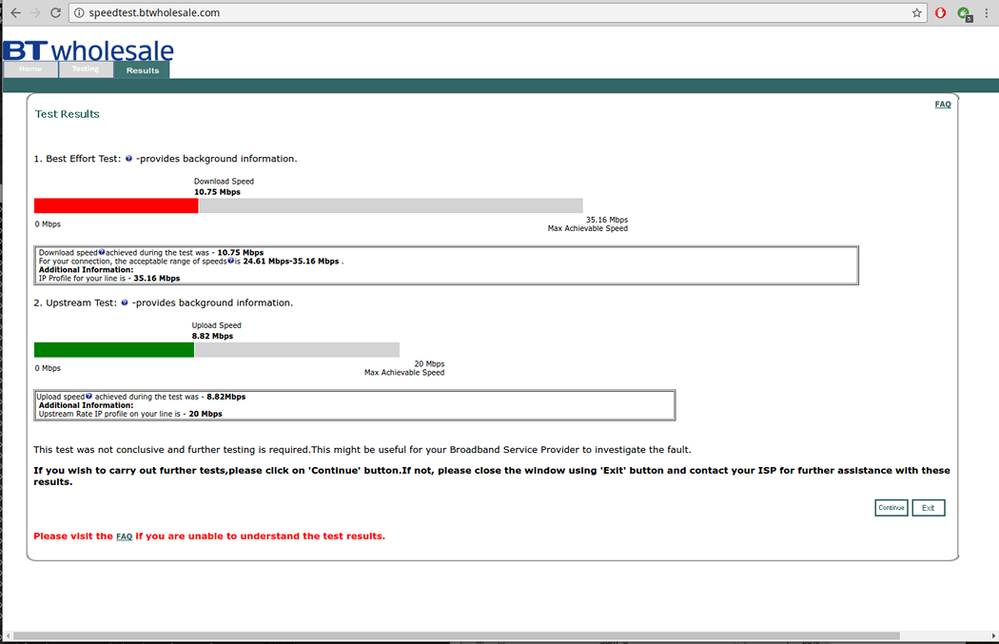cancel
Showing results for
Show  only  | Search instead for
Did you mean:Beginner
490 Views
Message 1 of 1

I've been monitoring my line sync speeds for a while now - they gradually reduced from mid-50Mbit upon signing up for Infinity 2, down to mid-40's to high 30's. I'm not too concerned, as they're relatively stable and I'm several hundred metres away from the cabinet, so was never expecting to get 70+.

```17:22:24, 28 Dec. DSL Link Up: Down Rate=36322Kbps, Up Rate=14071Kbps; SNR Margin Down=6.5dB, Up=6.1dB
16:07:06, 28 Dec. DSL Link Up: Down Rate=36917Kbps, Up Rate=13717Kbps; SNR Margin Down=6.2dB, Up=6.2dB
12:34:11, 23 Dec. DSL Link Up: Down Rate=36632Kbps, Up Rate=14042Kbps; SNR Margin Down=6.4dB, Up=6.1dB
10:54:50, 11 Dec. DSL Link Up: Down Rate=36322Kbps, Up Rate=13590Kbps; SNR Margin Down=6.4dB, Up=6.1dB
15:29:19, 10 Dec. DSL Link Up: Down Rate=36090Kbps, Up Rate=13687Kbps; SNR Margin Down=6.3dB, Up=6.1dB
11:39:39, 04 Dec. DSL Link Up: Down Rate=36219Kbps, Up Rate=13575Kbps; SNR Margin Down=6.4dB, Up=6.1dB
13:18:20, 27 Nov. DSL Link Up: Down Rate=36115Kbps, Up Rate=13448Kbps; SNR Margin Down=6.4dB, Up=6.1dB
13:11:01, 27 Nov. DSL Link Up: Down Rate=36167Kbps, Up Rate=13511Kbps; SNR Margin Down=6.3dB, Up=6.1dB
06:10:50, 18 Nov. DSL Link Up: Down Rate=35909Kbps, Up Rate=13201Kbps; SNR Margin Down=6.7dB, Up=6.1dB
08:20:41, 11 Nov. DSL Link Up: Down Rate=36452Kbps, Up Rate=13612Kbps; SNR Margin Down=6.5dB, Up=6.1dB
02:15:06, 09 Nov. DSL Link Up: Down Rate=40059Kbps, Up Rate=13497Kbps; SNR Margin Down=6.6dB, Up=6.1dB
15:29:40, 30 Oct. DSL Link Up: Down Rate=39625Kbps, Up Rate=13433Kbps; SNR Margin Down=6.5dB, Up=6.1dB
12:58:35, 22 Oct. DSL Link Up: Down Rate=39500Kbps, Up Rate=13336Kbps; SNR Margin Down=6.5dB, Up=6.1dB
18:18:43, 15 Oct. DSL Link Up: Down Rate=42548Kbps, Up Rate=13661Kbps; SNR Margin Down=6.2dB, Up=6.1dB
15:09:25, 15 Oct. DSL Link Up: Down Rate=43598Kbps, Up Rate=13590Kbps; SNR Margin Down=6.3dB, Up=6.1dB
10:40:24, 15 Oct. DSL Link Up: Down Rate=43471Kbps, Up Rate=13575Kbps; SNR Margin Down=6.4dB, Up=6.1dB
19:28:18, 08 Oct. DSL Link Up: Down Rate=43057Kbps, Up Rate=13672Kbps; SNR Margin Down=6.3dB, Up=6.1dB
13:03:05, 07 Oct. DSL Link Up: Down Rate=43662Kbps, Up Rate=13160Kbps; SNR Margin Down=6.3dB, Up=6.1dB
23:41:16, 06 Oct. DSL Link Up: Down Rate=43630Kbps, Up Rate=13485Kbps; SNR Margin Down=6.3dB, Up=6.1dB
15:58:18, 02 Oct. DSL Link Up: Down Rate=43566Kbps, Up Rate=13549Kbps; SNR Margin Down=6.2dB, Up=6.1dB
07:30:06, 19 Sep. DSL Link Up: Down Rate=43757Kbps, Up Rate=13272Kbps; SNR Margin Down=6.1dB, Up=6.3dB
22:31:40, 12 Sep. DSL Link Up: Down Rate=43121Kbps, Up Rate=13209Kbps; SNR Margin Down=6.2dB, Up=6.1dB
22:19:18, 29 Aug. DSL Link Up: Down Rate=43153Kbps, Up Rate=13433Kbps; SNR Margin Down=6.3dB, Up=6.1dB
19:50:15, 29 Aug. DSL Link Up: Down Rate=43216Kbps, Up Rate=13489Kbps; SNR Margin Down=6.2dB, Up=6.1dB
20:32:00, 16 Aug. DSL Link Up: Down Rate=44203Kbps, Up Rate=13444Kbps; SNR Margin Down=6.3dB, Up=6.1dB
20:25:12, 02 Aug. DSL Link Up: Down Rate=44457Kbps, Up Rate=13272Kbps; SNR Margin Down=6.3dB, Up=6.1dB
03:37:34, 28 Jul. DSL Link Up: Down Rate=46462Kbps, Up Rate=16012Kbps; SNR Margin Down=6.3dB, Up=4.5dB
04:28:00, 23 Jul. DSL Link Up: Down Rate=44776Kbps, Up Rate=12564Kbps; SNR Margin Down=6.3dB, Up=6.1dB
20:16:58, 19 Jul. DSL Link Up: Down Rate=41415Kbps, Up Rate=13265Kbps; SNR Margin Down=6.3dB, Up=6.1dB
10:39:19, 10 Jul. DSL Link Up: Down Rate=41519Kbps, Up Rate=13261Kbps; SNR Margin Down=6.2dB, Up=6.1dB
13:55:08, 09 Jul. DSL Link Up: Down Rate=41674Kbps, Up Rate=13209Kbps; SNR Margin Down=6.2dB, Up=6.1dB
13:41:58, 25 Jun. DSL Link Up: Down Rate=41725Kbps, Up Rate=13317Kbps; SNR Margin Down=6.2dB, Up=6.1dB
13:32:43, 11 Jun. DSL Link Up: Down Rate=41493Kbps, Up Rate=13015Kbps; SNR Margin Down=6.1dB, Up=6.1dB
16:43:52, 05 Jun. DSL Link Up: Down Rate=41984Kbps, Up Rate=13545Kbps; SNR Margin Down=6.2dB, Up=6.1dB
23:49:38, 02 Jun. DSL Link Up: Down Rate=42165Kbps, Up Rate=13403Kbps; SNR Margin Down=6.2dB, Up=6.1dB
19:34:41, 23 May. DSL Link Up: Down Rate=41958Kbps, Up Rate=13015Kbps; SNR Margin Down=6.1dB, Up=6.1dB
10:52:10, 19 May. DSL Link Up: Down Rate=42139Kbps, Up Rate=13190Kbps; SNR Margin Down=6.2dB, Up=6.1dB
10:41:34, 05 May. DSL Link Up: Down Rate=42397Kbps, Up Rate=13351Kbps; SNR Margin Down=6.2dB, Up=6.1dB```

As you can see, over 8-9 month period, it's relatively stable; some minor drop off towards the end of 2016, but as it's winter and cold and wet, I'd expect some degredation. No change in line quality though.

However, over the past few weeks (maybe months?) I've noticed that when I come to use downloads heavily (for example, big operating system upgrades which may take several gigs to download completely) that they almost always top out at 1MByte/sec, at best.

Every speed tester I try agrees within a megabit or two of each other:That's barely 25% of the line speed that I'm getting in actual download transfers and around 50-60% of my line speed in uploads.

Real life transfers fare no better - whether its downloading an Ubuntu iso from the UK mirrorservice at Kent, or data from my workstation or network drives at work (again, on JANet, so super fast uplinks) they get no higher than 1100-1200KBytes/sec.

So my line speed is still within the tolerances I have come to expect, but actual transfer speeds are dreadful. Any ideas?